# Let's Get Started

You will explore how to simplify expressions with exponents using the laws of exponents.

TEKS Standards and Student Expectations

A(11) Number and algebraic methods. The student applies the mathematical process standards and algebraic methods to rewrite algebraic expressions into equivalent forms. The student is expected to:

A(11)(B) simplify numeric and algebraic expressions using the laws of exponents, including integer and rational exponents.

Resource Objective(s)

Given verbal and symbolic descriptions of problems involving exponents, the student will simplify the expressions using the laws of exponents.

Essential Questions

What happens to the exponents when you multiply/divide powers with the same base?

What is the value of a term with an exponent of zero?

What happens to the expression when taking an exponential expression to a negative power?

Vocabulary

# The Laws of Exponents—The Product Law and The Quotient Law

The Laws of Exponents are composed of seven laws or rules that are applied when simplifying algebraic expressions and solving equations.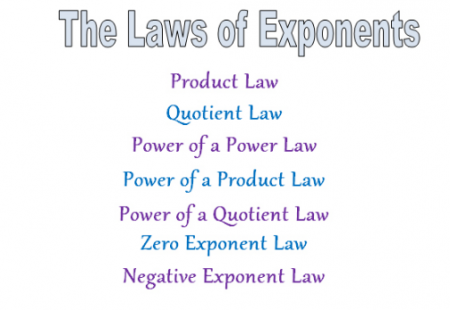Interactive Activity

Click on the following link to participate in an online interactive activity that will help explain the Product Law and Quotient Law. Then follow the directions below.

1. Click on “Bigger” in the upper right hand corner of the online interactive. (The following images are only illustrations. Use the provided link to complete the activity.)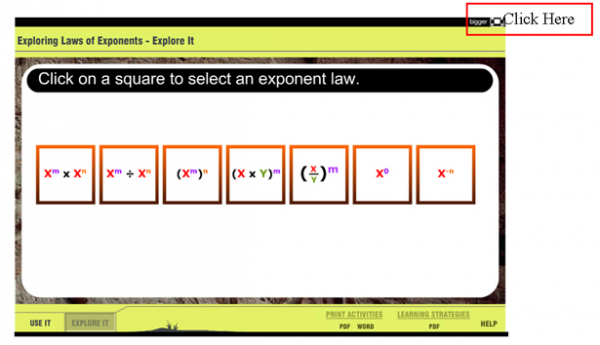2. Click on the first box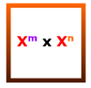to practice the Product Law.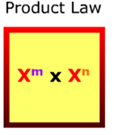3. The m-value exponent (purple), n-value exponent (orange), and x-variable are changed by clicking on a colored box on either the purple, orange, or red number line and moving your cursor to the left or right.

4. Select different values for m-, n-, and x–values. Each time a new value is selected, the Product Law is demonstrated, and the answer is shown at the bottom.

5. Practice until you understand the Product Law.

6. Click on the "X" next to the "Reset" button at the top right when done practicing to return to the original screen.

7. Click on the second box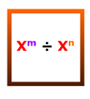to practice the Quotient Law.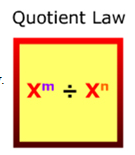8. Follow the previous directions to experiment until you understand the Quotient Law.

Answer the following three questions to check your understanding of the Product and Quotient Laws.  Click on the statement or problem to check your solution.

# The Power Laws—The Power of a Power Law, The Power of a Product Law, and The Power of a Quotient Law

Let's review the Power Laws: the Power of a Power Law, the Power of a Product Law, and the Power of a Quotient Law. Experiment with the Power Laws below using the same online interactive activity.

Interactive Activity

1. Click on “Bigger” in the upper right hand corner of the online interactive. (The following images are only illustrations. Use the provided link to complete the activity.)2. Click on the third boxto practice the Power of a Power Law.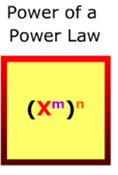3. The m-value exponent (purple), n-value exponent (orange), and x-variable are changed by clicking on a colored box on either the purple, orange, or red number line and moving your cursor to the left or right.

4. Select different values for m-, n-, and x–values. Each time a new value is selected, the Power of a Power Law is demonstrated, and the answer is shown at the bottom.

5. Practice until you understand the Power of a Power Law.

6. Click on the "X" next to the "Reset" button at the top right when done practicing to return to the original screen.

7. Click on the fourth box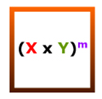to practice the Power of a Product Law.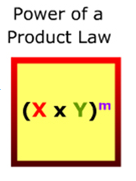9. Many students get confused about the difference between the Power of a Power Law and the Power of a Product Law. Make sure that you understand the difference between the two.

10. Click on the fifth boxto practice the Power of a Quotient Law.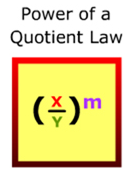Note: The Power of a Product Law and the Power of a Quotient Law only have one exponent.

Answer the following four questions to check your understanding of the Power of a Power Law, the Power of a Product Law, and the Power of a Quotient Law.  Click on the statement or problem to check your solution.

# The Zero Exponent Law

The Zero Exponent Law is considered the easiest exponent law. Experiment with the Zero Exponent Law below using the same online interactive activity.

Interactive Activity

1. Click on “Bigger” in the upper right hand corner of the online interactive. (The following images are only illustrations. Use the provided link to complete the activity.)2. Click on the sixth box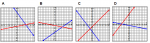to practice the Zero Exponent Law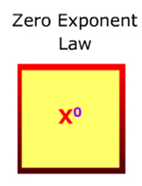.

3. The x-variable is changed by clicking on the box and moving your cursor to the left or right on the red number line.

Note: There is only zero for the exponent and only the x–values are changeable.

4. Practice until you understand the Zero Exponent Law.

5. Click on the "X" next to the "Reset" button at the top right when done practicing to return to the original screen.

Answer the following question. Click on the statement to check your solution.

# The Negative Exponent Law

The Negative Exponent Law is another easy law to remember. Explore and practice the Negative Exponent Law. Experiment using the same online interactive activity.

Interactive Activity

1. Click on “Bigger” in the upper right hand corner of the online interactive. (The following images are only illustrations. Use the provided link to complete the activity.)2. Click on the last boxto practice the Negative Exponent Law.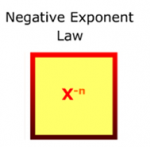3. The n-value (orange) and x-variable are changed by clicking on the box and moving your cursor to the left or right on the orange or red number line. Each time a new value is selected, the Negative Exponent Law is demonstrated, and the answer is shown at the bottom.

4. Practice until you understand the Negative Exponent Law.

5. When you are done practicing, click on the "X" next to the "Reset" button at the top right to return to the original screen.

Answer the following two questions. Click on the next slide to check your solution.

# Interactive Activity—Exponents and Dinosaurs

Interactive Activity

Now will use the Power Laws in a fun online interactive activity about dinosaurs.

1. Click on “Bigger” in the upper right hand corner of the online interactive. (The following images are only illustrations. Use the provided link to complete the activity.)2. Select Dig 1, 2, or 3.

3. Enter the dig and follow the instructions provided by the interactive. Clear the dirt, answer the questions, and build the dinosaur.

4. Click "Continue" and select Dig 4.

5. Enter the dig, clear the dirt, answer the questions, and build the dinosaur.

6. Practice until the all the Laws are understood or all the digs are completed.

Optional: Video segment. Assistance may be required. Watch this video showing a paleontologist applying the Laws of Exponents when working with measurement and scientific notation.